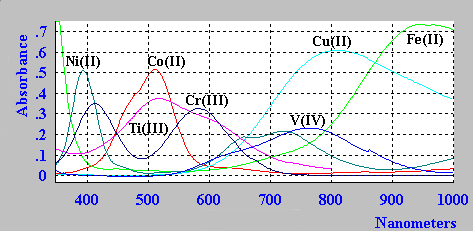# 20.9A: The Nephelauxetic Effect

$$\newcommand{\vecs}{\overset { \rightharpoonup} {\mathbf{#1}} }$$ $$\newcommand{\vecd}{\overset{-\!-\!\rightharpoonup}{\vphantom{a}\smash {#1}}}$$$$\newcommand{\id}{\mathrm{id}}$$ $$\newcommand{\Span}{\mathrm{span}}$$ $$\newcommand{\kernel}{\mathrm{null}\,}$$ $$\newcommand{\range}{\mathrm{range}\,}$$ $$\newcommand{\RealPart}{\mathrm{Re}}$$ $$\newcommand{\ImaginaryPart}{\mathrm{Im}}$$ $$\newcommand{\Argument}{\mathrm{Arg}}$$ $$\newcommand{\norm}{\| #1 \|}$$ $$\newcommand{\inner}{\langle #1, #2 \rangle}$$ $$\newcommand{\Span}{\mathrm{span}}$$ $$\newcommand{\id}{\mathrm{id}}$$ $$\newcommand{\Span}{\mathrm{span}}$$ $$\newcommand{\kernel}{\mathrm{null}\,}$$ $$\newcommand{\range}{\mathrm{range}\,}$$ $$\newcommand{\RealPart}{\mathrm{Re}}$$ $$\newcommand{\ImaginaryPart}{\mathrm{Im}}$$ $$\newcommand{\Argument}{\mathrm{Arg}}$$ $$\newcommand{\norm}{\| #1 \|}$$ $$\newcommand{\inner}{\langle #1, #2 \rangle}$$ $$\newcommand{\Span}{\mathrm{span}}$$$$\newcommand{\AA}{\unicode[.8,0]{x212B}}$$

The spectra of the aqua ions for some first row transition metal ions are shown below.For a much more detailed description of the interpretation of the spectra of first row transition metal ion complexes see the notes on the use of Tanabe-Sugano diagrams.

## Cr(III) - an example in more detail

For \9d^3\), $$d^8$$ octahedral and $$d^2$$, $$d^7$$ tetrahedral complexes, the above diagrams can be used to interpret the observed electronic absorption spectra.Figure 2

Take for example the $$\ce{Cr^{3+}}$$ aquo-ion $$\ce{ [Cr(H2O)6]^{3+}}$$. From the simplified Orgel diagram in Figure 2, three absorptions transitions are expected. In practice, the spectrum is found to contain three bands which occur at 17,000 cm-1, 24,000 cm-1 and 37,000 cm-1. Of which only two are shown in Figure 1.

$$μ_1$$ corresponds exactly to Δ (Delta) and since the lowest band is found at 17,000 cm-1 then this enables us to measure Δ directly from the spectrum.

The next band is found at 24,000 cm-1 and this can be equated to:

$μ_2=9/5Δ - x$

where $$x$$ is the configuration interaction between the T(F) state and the T(P) state of the same symmetry.

Since $$Δ$$ is 17,000 and μ2 is observed at 24,000 then x must be 6,600 cm-1.

The last band is seen at 37,000 cm-1 and here

$μ_3=6/5Δ + 15B + x \label{eq3}$

where $$B$$ is one of the RACAH parameters.

Solving Equation \ref{eq3} for $$B$$ gives a value of $$B=667\,cm^{-1}$$.

For the free Cr3+ ion, B is ~1030 cm-1 so that in the complex this term is reduced by ~2/3 of the free ion value.

A large reduction in B indicates a strong Nephelauxetic Effect. The Nephelauxetic Series is given by:

$\ce{F^{-} > H2O > urea > NH3 > en \~ C2O42^{-} > NCS^{-} > Cl^{-} ~ CN^{-} > Br^{-} > S2^{-} ~ I^-}$

Ionic ligands such as $$\ce{F^{-}}$$ give a small reduction in $$B$$, while covalently bonded ligands such as $$\ce{I^{-}}$$ give a large reduction of $$B$$.